# Hypothesis Testing

### Sarah Ogilvie

#### Analytic Strategy

To explore hypotheses about frame use between motivational conditions, analysis of variance techniques were used, with the dependent variables being transformed proportions of frame use in each case. In comparing frame use between conditions, the main focus was upon differences between cooperative and competitive conditions, although some exploratory analysis was conducted across the three conditions. An alpha level of 0.05 was used for all tests of significance.

#### Cooperative Versus Competitive Motivation Condition

H1: Negotiators in the cooperative motivation condition are more likely to express cooperatively-oriented frames -- cooperative aspiration, cooperative outcome and inclusive process frames -- than negotiators in the competitive motivation condition.

The results of a single-factor MANOVA with the three cooperative frame types as dependent variables (cooperative aspiration, cooperative outcome and inclusive process frames) and condition (cooperative versus competitive) as a between-subject factor indicated that the cooperative set of frames was not used more in the cooperative, relative to the competitive, condition, F (3,25) = 0.781, p = .516, â•¬Ã€â”¬â–“ = .086. The average use of cooperatively-oriented frames in each condition is depicted in Figure 2. Results of univariate tests are reported subsequently in Section 5.4.1.3.1.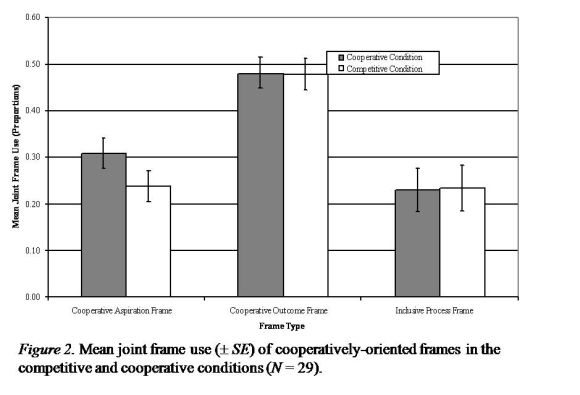H2: Competitively motivated negotiators will use more competitively-oriented frames (competitive aspiration, competitive outcome and non-inclusive process frames) than will cooperatively motivated negotiators.

The results of a single-factor MANOVA with the three frame types as dependent variables and condition (cooperative versus competitive) as an independent variable, showed that competitively-oriented frames were not used significantly more in the competitive than cooperative condition F (3, 25) = 2.824, p = 0.059, â•¬Ã€â”¬â–“ = 0.253. Nevertheless, a trend toward greater use of competitively-oriented frames by competitors was found and can be explained by the observation that the competitors used more competitive aspiration and non-inclusive process frames than the cooperators, despite using fewer competitive outcome frames. Mean use of competitively-oriented frames between competitors and cooperators is presented in Figure 3.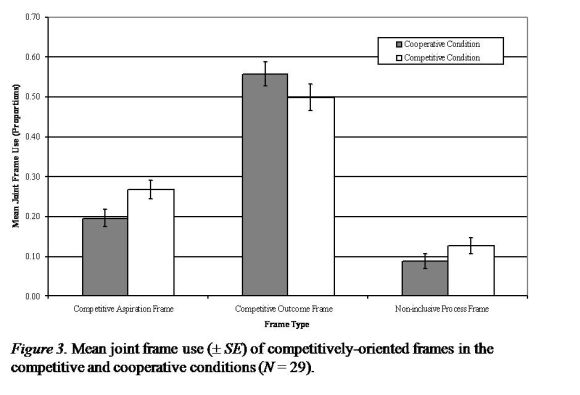H3: Negotiators in the competitive condition will use more power/rights-based justification frames than negotiators in the cooperative condition.

An ANOVA was conducted to test the difference in frame use between competitively motivated negotiators (M = 0.261, SD = 0.120) and cooperatively motivated negotiators (M = 0.182, SD = 0.103). Marginal support was found for this hypothesis, F (1, 28) = 3.638, p = .067, â•¬Ã€â”¬â–“ = .119.

#### Comparison of Frame Use in Same Versus Mixed Motivation Conditions

Although no predictions were made about differences in frame use between same and mixed motivation conditions, some exploratory analyses in this area were undertaken (Exploratory Hypothesis 1). To compare the use of frames between same and mixed motivation conditions, a single-factor MANOVA was conducted in which the eight frames were the dependent variables and condition (same versus mixed motivation) constituted the between subject factor. For the purposes of this analysis both pairs of cooperators and pairs of competitors were assigned to the same motivational orientation group. Results showed no difference in the use of frames between same and mixed motivation dyads, F (8, 35) = 0.629, p = .748, â•¬Ã€â”¬â–“ = .126.

#### Tests of univariate frame use between conditions.

Although there were indicative trends, in testing subsets of frame use between conditions (Hypotheses 1, 2, 3 and Exploratory Hypothesis 1), no significant differences were found. Despite the risk of inflated Type I error, univariate trends in frame use between conditions were examined. These trends have been summarised in Table 5.

Frame type Difference in use between cooperative and competitive conditions (N = 29) Difference in use between same motivation and mixed motivation conditions (N = 44)
Cooperative Aspiration F (1,27) = 2.350, p = .137 F (1,42) = 0.118, p = .733
Competitive Aspiration F (1,27) = 5.454, p = .027* F (1,42) = 0. 645, p = .426
Cooperative Outcome F (1,27) = 0.002, p = .961 F (1,42) = 0.529, p = .471
Competitive Outcome F (1,27) = 1.675, p = .207 F (1,42) = 0.096, p = .758
Substantive Issues F (1,27) = 1.466, p = .237 F (1,42) = 0.519, p = .475
Inclusive Process F (1,27) = 0.003, p = .954 F (1,42) = 0.664, p = .420
Non-inclusive Process F (1,27) = 2.021, p = .167 F (1,42) = 4.364, p = .043*
Power/Rights-based Justification F (1,27) = 3.638, p = .067 F (1,42) = 0.511, p = .479

* p < .05.

As Table 5 shows, only use of the competitive aspiration frame was found to differ between competitors and cooperators, with competitors using this frame more frequently (cooperators: M = .196, SD = .082; competitors: M = .268, SD = .084). Table 5 also reveals that the use of non-inclusive process frames differed between mixed and same motivation conditions, with negotiators in the mixed condition using this frame more frequently (mixed motivation condition: M = .161, SD = .094, same motivation conditions: M = .107, SD = .074).

#### Frame Use Within the Mixed Motivation Condition

H4: In mixed dyads, competitively-oriented frames will be more frequently used than will cooperatively-oriented frames.

Hypothesis 4 would be supported if the subset of competitively-oriented frames (competitive aspiration, competitive outcome and non-inclusive process) was used more often than the subset of cooperatively-oriented frames (cooperative aspiration, cooperative outcome and inclusive process frames). To test this hypothesis, the six frames were classified according to their orientation (cooperative or competitive) and frame type (aspiration, outcome or process). Frame use was then analysed using a 2 orientation x 3 frame type doubly multivariate MANOVA in which frame orientation was the repeated measures factor and aspiration, outcome and process represented the three measures. Results showed that negotiators did not employ competitively-oriented frames more frequently than cooperatively-oriented frames, F (3, 12) = 1.140, p = .372, â•¬Ã€â”¬â–“ = .222. The average use of these six frames within mixed dyads is shown in Figure 4.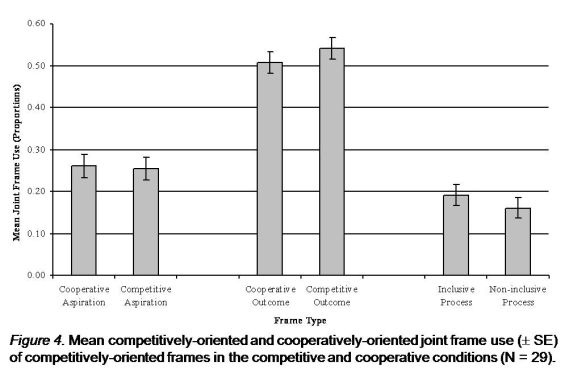#### Data Treatment

To analyse shifts in frame use across time, the negotiations were divided into two equal-length segments according to the number of uninterrupted negotiator speaking turns. A more fine-grained analysis of time effects was not possible because of the low frequency in use of some of the frames. These two equal length segments are referred to subsequently as Time 1 and Time 2 respectively.

#### Analytic Strategy: Changes in Frame Use Over Time

The second set of hypotheses addressed both shifts in frame use across time and the types of outcomes achieved given certain shifts in frame use (Hypotheses 5 - 15). These hypotheses focussed upon frame use within motivational conditions rather than between conditions. To test for frame shifts within conditions, repeated measures analyses were conducted.

To reiterate, the following figures describe patterns in frame shifts. Figure 5 shows convergence in the use of a frame is defined as instances in which the difference in frame use between negotiators within a dyad at Time 1 is larger than at Time 2. A smaller difference between negotiators in the use of a particular frame at Time 2 indicates that negotiators are using that frame to a more similar extent in the second half of the negotiation.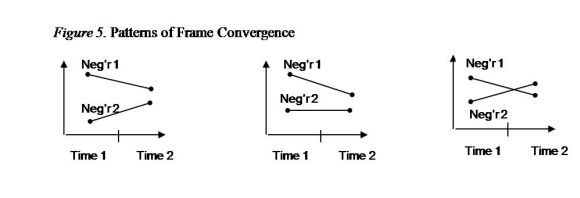Frame divergence describes the opposite pattern: larger differences in frame use at Time 2 indicate that the frame is used to a less similar extent in the second half of the negotiation.Frame maintenance is defined as instances in which there is no difference in the use of a frame between negotiators across time.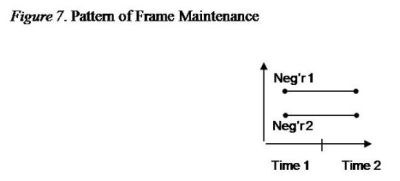Frame shift equivalence occurs when differences in frame use are maintained across time. Unlike frame maintenance, frame shift equivalence indicates no difference in use between negotiators across time, but a simultaneous increase or decrease in joint frame use over time. The third possibility for frame shift equivalence is for one party to increase its frame use by the same amount that the other party decreases its frame use.Frame difference scores were used to test for frame convergence, divergence and maintenance. These scores indicate the degree to which the frequency of frame use differed between members of a dyad. For each dyad, absolute frame difference scores were calculated for Time 1 and Time 2.

A larger difference at Time 1 than at Time 2 indicated convergence in frame use and a larger difference at Time 2 than at Time 1 indicated divergence in frame use. No difference across time indicated either maintenance in the use of frames or frame shift equivalence. To establish frame shift equivalence, frame maintenance was first established. Joint frame scores were then entered into a repeated measures design to determine whether there was a main effect for time and, if so, whether frame use simultaneously increased or decreased over time.

#### Analytic Strategy: The Relationship Between Frame Shifts and Joint Outcomes

The relationship between frame shifts and joint outcomes was investigated by regressing joint outcomes -- total number of points obtained by the two negotiators -- onto a measure that gauges the extent of shift in frame use over time. This measure was created by converting the frame difference scores at Time 1 and Time 2 into one set of scores. For each frame, the measure was calculated by subtracting the absolute value of the frame difference score at Time 1 from the score at Time 2. This measure of the change in frame difference generated a set of eight new variables -- one per frame -- that represented the degree to which frames converged over time.

Regression analyses were conducted only for the cases in which shifts in frame use were found. Because the hypotheses concerned relationships within conditions, individual regression analyses were conducted within each motivational condition.

#### Test of Hypotheses: Cooperative Motivation Condition

H5: Convergence in the use of competitively-oriented frames (competitive aspiration, competitive outcome and non-inclusive process frames) in the cooperative condition will be associated with more integrative outcomes (high joint gain).

In testing this hypothesis, two stages were planned: first, convergence in the set of competitively-oriented frames needed to be established and second, on finding convergence, the relationship between convergence and joint outcome would then be tested. To determine convergence in frame use, a 2 (Time 1 versus Time 2) x 3 (competitive aspiration, competitive outcome, non-inclusive process frames) repeated measures MANOVA was conducted, with time the within subject factor and the three frame types the dependent variables. Results indicated that competitively-oriented frames did not converge significantly over time despite a trend in the expected direction, F (3, 12) = 3.192, p = .063, â•¬Ã€â”¬â–“ = .444. Because results trended towards convergence, univariate results were examined and use of the non-inclusive process frame was found to converge across time F (1, 14) = 8.628, p = .011, â•¬Ã€â”¬â–“ = .381. Figure 5 shows the mean differences in frame use across time for all three frames.Note: Convergence is indicated by lower values at Time 2.

Because the non-inclusive process frame was found to significantly converge over time, the relationship between convergence and joint outcome was tested. The measure of joint outcome was regressed onto the measure of non-inclusive process frame convergence. Joint outcome was unrelated to convergence, R2 = 0.004, Adj R2= -0.073, SE = 1439.920, p = .832.

#### Test of Hypotheses: Competitive Motivation Condition

H6: In the competitive motivation condition, the use of power/rights justification-based frames will converge over time.

To test this hypothesis, a repeated measures ANOVA was used with time (Time 1 versus Time 2) the within subject factor and the power/rights-based justification frame the dependent variable. Results indicated that use of this frame did not converge over time; F (1,13) = 2.503, p = .138, â•¬Ã€â”¬â–“ = .161, Time 1 difference: M = .0621, SD = .021, Time 2 difference: M = .146, SD = .046.

H8: In the competitive condition, the convergence of cooperatively-oriented frames will be associated with more integrative outcomes (high joint gain).

To test Hypothesis 8, two stages were planned: after establishing whether there was convergence in the subset of cooperatively-oriented frames, the relationship between convergence and joint outcome would then be tested. To determine convergence in frame use, a 2 (Time 1, Time 2) x 3 (cooperatively-oriented frames) repeated measures MANOVA was conducted, with time the within subject factor and cooperatively-oriented frames the dependent variables. The set of cooperatively-oriented frames did not vary with time, F (3,11) = 0.168, p = .915, â•¬Ã€â”¬â–“ = .044, which suggests that negotiators had not converged. Hence, there was no further testing of this hypothesis. Trends in the average differences in cooperatively-oriented frame use over time are shown in Figure 6.Note: Trends towards convergence are indicated by lower values at Time 2; higher values, divergence.

H9: Convergence in the use of substantive issues frames in the competitive condition will be associated with more integrative outcomes (high joint gain).

To demonstrate convergence in the use of this frame, a repeated measures ANOVA was conducted, with the within subject factor being time and the substantive issues frame the dependent variable. The hypothesis was not supported, F (1, 13) = 1.567, p = .233, â•¬Ã€â”¬â–“ = .108; Time 1 difference: M = .138, SD = .043, Time 2 difference: M = .074, SD = .020, and hence, this hypothesis was not tested further.

#### Test of Hypotheses: Mixed Motivation Condition

H10: In the mixed motivation condition, the cooperator will shift towards the competitor's use of frames.

H11 (Linked to H10): In contrast to the cooperator, the frames used by the competitor will be maintained during the course of the negotiation.

These hypotheses would be supported if the cooperator's frames converge towards the competitor's frame, and if the competitor's frame use was maintained (i.e., the cooperator is responsible for the convergence). To test Hypothesis 10, the strategy was first to determine whether there was convergence in frame use across time and, if so, to determine which of the negotiators was responsible for the convergence.

To test for convergence, a 2 (Time 1 versus Time 2) x 8 (frame type) repeated measures MANOVA design was conducted with time the within participants factor and the eight frame types the dependent variables. Results indicated that frames did not converge over time, F (8,7) = 1.508, p = .301, â•¬Ã€â”¬â–“ = .633. Because frame use was maintained over time, Hypothesis 13 was not tested.

H13: In contrast to Hypotheses 10 and 11, negotiators in the mixed motivation condition are predicted to shift to adopt a set of frames that differs from frames they initially adopt in the negotiation.

This hypothesis would be supported if the frequency pattern of frame use at Time 1 differed from that at Time 2. In testing this hypothesis, the joint frame scores data was used because the focus was upon the frames that negotiators used jointly and not upon the differences in frame use between negotiators. Figure 7 shows the frequency of use for all frames across time in the mixed motivation condition.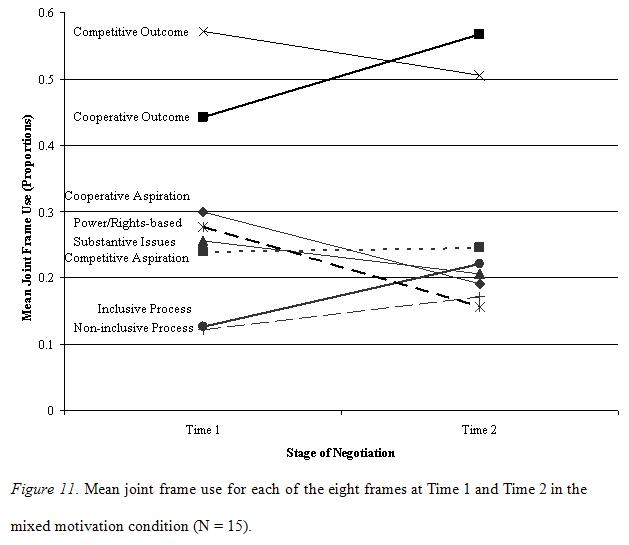Note: Points in time indicate changes in joint frame use, not changes in difference in frame use.

To test whether any of these shifts in joint frame use across time were significant, a 2 (Time 1 versus Time 2) x 8 (frame type) repeated measures MANOVA was conducted with time the within participants factor and joint frame scores the dependent variables. Results indicated a trend towards significant shifts in the joint use of frames, F (8,7) = 2.885, p = .090, â•¬Ã€â”¬â–“ = .767. Examination of univariate trends showed that the following frames were found to increase over time: cooperative outcome frame, F (1, 14) = 21.062, p < .001, â•¬Ã€â”¬â–“ = .601 and of the inclusive process frame, F (1, 14) = 7.530, p = .016, â•¬Ã€â”¬â–“ = .350. In contrast, use of the cooperative aspiration frame, F (1, 14) = 10.076, p = .007, â•¬Ã€â”¬â–“ = .419; competitive outcome frame, F (1, 14) = 5.385, p = .036, â•¬Ã€â”¬â–“ = .278; and the power/rights-based justification frame, F (1, 14) = 6.182, p = .026, â•¬Ã€â”¬â–“ = .306, were found to decrease over time. Table 6 shows the pattern of frame use at Time 1 and Time 2 and specifies which frames significantly increased or decreased in joint use across time.

#### Table 6

Joint Frame Occurrence at Time 1 and Time 2, Listed from the Most Frequently Used to the Least Frequently Used (N = 15)
Pattern of Frame Occurrence Time 1 Time 2
Occurred Most 1 Competitive Outcome Cooperative OutcomeI
2 Cooperative Outcome Competitive OutcomeD
3 Cooperative Aspiration Competitive Aspiration
4 Power/Rights-based Justification Inclusive ProcessI
5 Substantive Issues Substantive Issues
6 Competitive Aspiration Cooperative AspirationD
7 Inclusive Process Non-inclusive Process
Occurred Least 8 Non-inclusive Process Power/Rights-based JustificationD

I significant increase over time
D significant decrease over time

These results revealed that, for mixed motivation negotiators, the pattern of joint frame use changed over time, and significantly changed over time for five of the eight frames.

#### The relationship between joint frame use and outcomes in the mixed motivation condition.

On establishing differences across time in the pattern of joint frame use, the relationship between shifts in joint frame use and negotiated outcomes was investigated using a regression analysis. To preserve the continuous nature of the outcome variable, the joint frame scores data at Time 1 and Time 2 were converted into one set of variables to represent the change in joint frame use across time. Specifically, joint frame scores at Time 1 were subtracted from joint frame scores at Time 2, which generated a joint frame shift measure for each of the eight frames, wherein higher scores indicated an increase in joint frame use over time and lower scores indicated a decrease.

To test the relationship between frame shift and outcomes in the mixed motivation condition, the measure of joint outcomes was regressed onto the joint frame shift measures for the five frames that were found to have changed in use over time (cooperative aspiration, cooperative outcome, competitive outcome, inclusive process and power/rights-based justification frames).

Results of the regression analysis indicated that there was a trend towards the set of frames predicting joint outcomes, Râ”¬â–“ = 0.600, Adj Râ”¬â–“= 0.378, SE = 877.902, p = .096. In particular, the shift in the use of the competitive outcome frame was found to be a significant predictor of joint outcomes as Table 7 demonstrates.

#### Table 7

Individual Frame Predictors of Joint Outcomes in the Mixed Motivation Condition (N = 15)
Frame Type B Std. Error â•¬â–“ T Sig.
Cooperative Aspiration 4367.101 3061.258 .426 1.427 .187
Cooperative Outcome 4871.683 3173.415 .365 1.535 .159
Competitive Outcome -17306.480 6102.135 -.751 -2.836 .020*
Inclusive Process 4715.505 2869.916 .378 1.643 .135
Power/Rights-based Justification -1785.789 2090.925 -.233 -.854 .415

* p < 0.05

Recalling that higher scores reflect an increase in joint frame use and lower scores a decrease in use, these findings indicate that a decrease in joint use of the competitive outcome frame was predictive of more integrative outcomes.

#### Additional Analyses: Frame Shift Equivalence

The primary reason for investigating frame shifts over time was to ascertain whether frames converged, diverged, or were maintained. When differences in frame use were maintained, a second analysis was conducted to determine whether frame use shifted in an equivalent manner. This analysis was undertaken in the same way as the analysis of convergence and divergence but used joint frames scores rather than the frame difference scores as dependent variables. Whereas the latter indexed changes in the differences between the negotiators over time, the former indexed changes in joint frame use.

#### Test of Frame Shift Equivalence: Cooperative Motivation Condition

In the cooperative motivation condition, the use of cooperatively-oriented frames neither converged nor diverged over time. A test for frame shift equivalence was therefore conducted. A 2 (Time 1 versus Time 2) x 3 (cooperatively-oriented frames) repeated measures MANOVA was conducted with time the within participants factor and joint frames the dependent variables. The results of this analysis indicated that joint use of the cooperatively-oriented set of frames did not significantly change over time within cooperatively motivated dyads, F (3,12) = 0.990, p = .430, â•¬Ã€â”¬â–“ = .198.

The set of competitively-oriented frames was also maintained over time in the cooperative condition. Testing for frame shift equivalence, a 2 (Time 1 versus Time 2) x 3 (competitively-oriented frames) repeated measures MANOVA was conducted with time the within participants factor and joint frames the dependent variables. Results demonstrated a trend towards joint use of the competitively-oriented frames decreasing over time, F (3,12) = 2.779, p = .087, â•¬Ã€â”¬â–“ = .410. Analysis of univariate effects showed that of the three frames included in the analysis, joint use of non-inclusive process frames significantly decreased over time, F (1,14) = 7.473, p = .016, â•¬Ã€â”¬â–“ = .348; Time 1: M = .106, SD = .082, Time 2: M = .039, SD = .068.

The relationship between use of non-inclusive process frames and joint outcomes was then tested. The results indicated that joint shift in the use of this frame did not predict joint outcomes, R2 = 0.001, Adj R2= -0.075, SE = 1291.09, p = .897.

#### Test of Frame Shift Equivalence: Competitive Motivation Condition

In the competitive motivation condition, neither convergence nor divergence was detected in the use of the power/rights-based justification frame. A test of frame shift equivalence was conducted on this variable using a repeated measures ANOVA. In competitively motivated dyads, joint use of the power/rights-based justification frame did not significantly increase or decrease over time, F (1, 13) = 0.265, p = .615, â•¬Ã€â”¬â–“ = .020.

The cooperatively-oriented set of frames was also found to be maintained over time. A 2 (Time 1, Time 2) x 3 (cooperatively-oriented frames) repeated measures MANOVA was conducted with time the within participants factor and joint frames the dependent variables. Within competitively motivated dyads, joint use of cooperatively-oriented frames significantly changed over time, F (3, 11) = 5.354, p = 0.016, â•¬Ã€â”¬â–“ = .594. Of the three cooperatively-oriented frames univariate tests revealed that joint use of the cooperative outcome frame significantly increased over time, F (1, 13) = 11.852, p = 0.004, â•¬Ã€â”¬â–“ = .477; Time 1: M = .407, SD = .094, Time 2: M = .542, SD = .125.

To test the relationship between frame shift equivalence of the cooperatively-oriented frames and outcomes, joint outcomes were regressed onto the joint frame shift scores for this set of frames. The results indicated that shifts in the use of these frames did not predict joint outcomes, R2 = 0.426, Adj R2= 0.254, SE = 860.57, p = .121.

Finally, because use of substantive issues frames was found to be maintained across time in the competitive condition, the pattern of frame shift equivalence was tested. Using a repeated measures ANOVA with the within participants factor being time and the dependent variable being the substantive issues frame, a decrease over time in the joint use of substantive issues frames was found, F (1, 13) = 23.232, p < .001, â•¬Ã€â”¬â–“ = .641; Time 1: M = .358, SD = .058, Time 2: M = .188, SD = .131.

A regression of joint outcomes on the substantive issues frame showed a non-significant relationship between use of this frame and joint outcomes, R2 = 0.246, Adj R2= 0.183, SE = 900.67, p = .071 (â•¬â–“ = .496). Increased joint use of the substantive issues frame was related to more integrative outcomes; more specifically, as joint use of this frame decreased over time, joint use of the substantive issues frame in the competitive condition was related to more distributive outcomes or to lower joint gain.